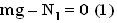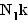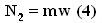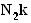## Tuesday, August 14, 2007

### Irodov Problem 1.79Let the mass of each of the two bodies be m and let the tension in the string be T. Further let the minimum acceleration required to keep mass from moving be w. Now let us consider the forces acting on each of the masses.

Forces on body 1: There are two forces acting on this body in the vertical direction, i) the normal reaction from the body A and ii) the force of gravity as shown in the figure. There is no acceleration of this mass along the vertical direction. Hence we have,In the horizontal direction, there are two forces acting on the mass, i) the tension in the string T and ii) the force of friction given by. The mass accelerates at a rate w. Hence, we have,From (2) and (1) we have,

T - mgk = mw (3)

Forces on body 2: There is only one force acting on the body in the horizontal direction - the normal reaction from the surface which is responsible for pushing it forward at a rate w. Thus, we have,There are three forces acting on the body in the vertical direction, i) the force of friction opposing the motion of body 2, ii) the force of gravity pulling it down mg and iii) tension T pulling it up. The mass does not accelerate along this direction. Hence , we have,From (4) and (5) we have,

mg - T - mwk = 0 (6)
From (3) and (6) we have,

mg - mgk - mw - mwk = 0 or,
g(1-k) = w(1+k) or,#### 1 comment:

ASIF said...

VERY GOOD FAR BETTER THAN ARIHANTS &ABHAY SINGH SOLUTIONS MOHAMMED ASIF (PHYSICS IIT FACULTY INSIGHT HYDERABAD)&GUEST FACULTY FOR NEW VISION JR COLLEGE( S.R NAGAR)